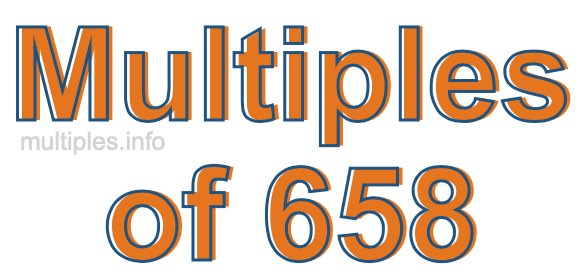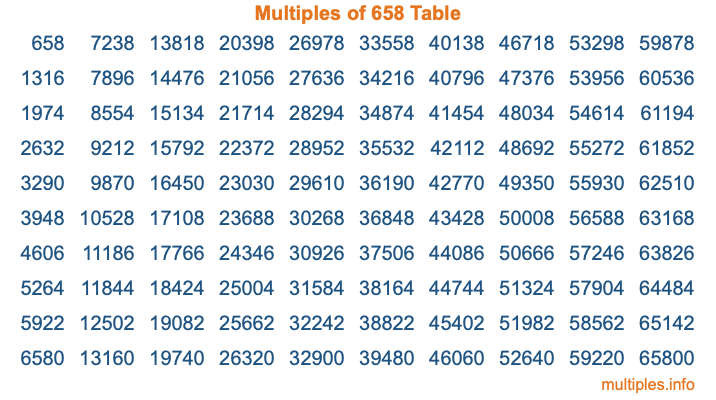Multiples of 658Welcome to the Multiples of 658 page. Here we will first teach you everything you will ever need to know about the multiples of 658, and then give you a study guide summary of everything we taught you to make sure you remember it all. Use this page to look up facts and learn information about the multiples of 658. This page will make you a multiples of six hundred fifty-eight expert!

Definition of Multiples of 658
Multiples of 658 are all the numbers that when divided by 658 equal an integer. Each of the multiples of 658 are called a multiple. A multiple of 658 is created by multiplying 658 by an integer.

Therefore, to create a list of multiples of 658, you start with 1 multiplied by 658, then 2 multiplied by 658, then 3 multiplied by 658, and so on for as long as you want. Thus, the list of the first five multiples of 658 is 658, 1316, 1974, 2632, and 3290. To see a larger list of multiples of 658, see the printable image of Multiples of 658 further down on this page. We also have a category where you can choose any nth multiple of 658.

Multiples of 658 Checker
The Multiples of 658 Checker below checks to see if any number of your choice is a multiple of 658. In other words, it checks to see if there is any number (integer) that when multiplied by 658 will equal your number. To do that, we divide your number by 658. If the the quotient is an integer, then your number is a multiple of 658.

Is  a multiple of 658?

Least Common Multiple of 658 and ...
A Least Common Multiple (LCM) is the lowest multiple that two or more numbers have in common. This is also called the smallest common multiple or lowest common multiple and is useful to know when you are adding our subtracting fractions. Enter one or more numbers below (658 is already entered) to find the LCM.

Check out our LCM Calculator if you need more details about the Least Common Multiple or if you need the LCM for different numbers for adding and subtraction fractions.

nth Multiple of 658
As we stated above, 658 is the first multiple of 658, 1316 is the second multiple of 658, 1974 is the third multiple of 658, and so on. Enter a number below to find the nth multiple of 658.

th multiple of 658

Multiples of 658 vs Factors of 658
658 is a multiple of 658 and a factor of 658, but that is where the similarities end. All postive multiples of 658 are 658 or greater than 658. All positive factors of 658 are 658 or less than 658.

Below is the beginning list of multiples of 658 and the factors of 658 so you can compare:

Multiples of 658: 658, 1316, 1974, 2632, 3290, etc.

Factors of 658: 1, 2, 7, 14, 47, 94, 329, 658

As you can see, the multiples of 658 are all the numbers that you can divide by 658 to get a whole number. The factors of 658, on the other hand, are all the whole numbers that you can multiply by another whole number to get 658.

It's also interesting to note that if a number (x) is a factor of 658, then 658 will also be a multiple of that number (x).

Multiples of 658 vs Divisors of 658
The divisors of 658 are all the integers that 658 can be divided by evenly. Below is a list of the divisors of 658.

Divisors of 658: 1, 2, 7, 14, 47, 94, 329, 658

The interesting thing to note here is that if you take any multiple of 658 and divide it by a divisor of 658, you will see that the quotient is an integer.

Multiples of 658 Table
Below is an image of the first 100 multiples of 658 in a table. The table is in chronological order, column by column. The first column has the first ten multiples of 658, the second column has the next ten multiples of 658, and so on.The Multiples of 658 Table is also referred to as the 658 Times Table or Times Table of 658. You are welcome to print out our table for your studies.

Negative Multiples of 658
Although not often discussed or needed in math, it is worth mentioning that you can make a list of negative multiples of 658 by multiplying 658 by -1, then by -2, then by -3, and so on, to get the following list of negative multiples of 658:

-658, -1316, -1974, -2632, -3290, etc.

Multiples of 658 Summary
Below is a summary of important Multiples of 658 facts that we have discussed on this page. To retain the knowledge on this page, we recommend that you read through the summary and explain to yourself or a study partner why they hold true.

There are an infinite number of multiples of 658.

A multiple of 658 divided by 658 will equal a whole number.

658 divided by a factor of 658 equals a divisor of 658.

The nth multiple of 658 is n times 658.

The largest factor of 658 is equal to the first positive multiple of 658.

658 is a multiple of every factor of 658.

658 is a multiple of 658.

A multiple of 658 divided by a divisor of 658 equals an integer.

658 divided by a divisor of 658 equals a factor of 658.

Any integer times 658 will equal a multiple of 658.

Multiples of a Number
Here you can get the multiples of another number, all with the same attention to detail as we did for multiples of 658 on this page.

Multiples of
Multiples of 659
Did you find our page about multiples of six hundred fifty-eight educational? Do you want more knowledge? Check out the multiples of the next number on our list!